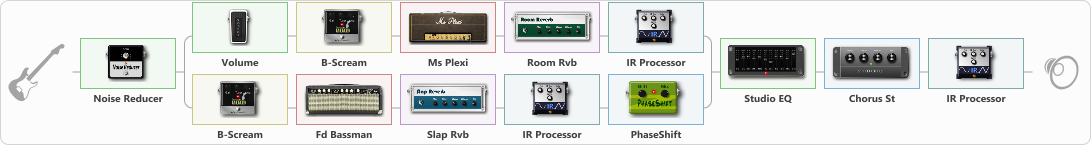# Kevlar Suit

Discussion in 'ToneLib-GFX presets' started by Neronte, Nov 25, 2022.

1. Kevlar Suit

Preset name: Kevlar Suit

Effects chain:Effect: "Noise Reducer" (Dynamics / Filter), active - "yes"
{
"Sens" = 74
"Mode" = Hard
}

Effect: "Splitter" (Dynamics / Filter)
{
"A-Bypass" = Off
"A-Pan" = 0
"A-Level" = 60
"B-Bypass" = Off
"B-Pan" = 0
"B-Level" = 60
"Width" = 0

'A' branch:
{

Effect: "Volume" (Dynamics / Filter), active - "yes"
{
"Pedal Pos." = 25
"Pan" = 0
}

Effect: "B-Scream" (Overdrive / Distortion), active - "yes"
{
"Drive" = 100
"Tone" = 100
"Level" = 60
}

Effect: "Ms Plexi" (Amp simulators), active - "yes"
{
"Gain" = 100
"Bass" = 100
"Middle" = 70
"Treble" = 37
"Presence" = 100
"Master" = 100
"Level (dB)" = 0
}

Effect: "Room Rvb" (Reverberation), active - "yes"
{
"Time" = 1.0
"PreDelay" = 0
"LoDamp" = 46
"HiDamp" = 25
"Mix" = 9
}

Effect: "IR Processor" (Cabinets), active - "yes"
{
"IR" = Marshall Amplification
"Low Cut (Hz)" = 73
"Hi Cut (kHz)" = 11.0
"Mix" = 100
"Level (dB)" = 0
}
}
'B' branch:
{

Effect: "B-Scream" (Overdrive / Distortion), active - "yes"
{
"Drive" = 58
"Tone" = 100
"Level" = 60
}

Effect: "Fd Bassman" (Amp simulators), active - "yes"
{
"Gain" = 100
"Bass" = 100
"Middle" = 50
"Treble" = 50
"Presence" = 100
"Master" = 50
"Level (dB)" = 0
}

Effect: "Slap Rvb" (Reverberation), active - "yes"
{
"Time" = 1.0
"PreDelay" = 0
"LoDamp" = 37
"HiDamp" = 54
"Mix" = 10
}

Effect: "IR Processor" (Cabinets), active - "yes"
{
"IR" = YA BMAN 410 P10Q 57-1
"Low Cut (Hz)" = 34
"Hi Cut (kHz)" = 10.5
"Mix" = 100
"Level (dB)" = 0
}

Effect: "PhaseShift" (Modulation / Sfx), active - "yes"
{
"Shift" = 50
"Mix" = 100
}
}
}

Effect: "Studio EQ" (Dynamics / Filter), active - "yes"
{
"31 Hz" = 15
"62 Hz" = 15
"125 Hz" = 0
"250 Hz" = 0
"500 Hz" = 0
"1 kHz" = 0
"2 kHz" = 0
"4 kHz" = 0
"8 kHz" = 0
"16 kHz" = 15
"Level (dB)" = 0
}

Effect: "Chorus St" (Modulation / Sfx), active - "yes"
{
"Speed" = 1.9
"Depth" = 31
"Center" = 4.4
"Mix" = 25
}

Effect: "IR Processor" (Cabinets), active - "yes"
{
"IR" = Rev IR Sample5
"Low Cut (Hz)" = 66
"Hi Cut (kHz)" = 20.0
"Mix" = 2
"Level (dB)" = 0
}

Note: You will need to download and install the ToneLib-GFX software to use the preset.

#### Attached Files:

• ###### Kevlar_Suit.tlgfx
File size:
77.4 KB
Views:
2,549
Bruno Pureza likes this.